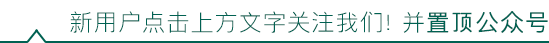# 深入理解 PHP7 内核之 OBJECTwww.laruence.com/2020/03/23/5605.html

### PHP5

PHP5中，对象的定义如下：

typedef struct _zend_object {
zend_class_entry *ce;
HashTable *properties;
zval **properties_table;
HashTable *guards;
} zend_object;


zend_object(以下简称object)在PHP5中其实是一种相对特殊的存在， 在PHP5中，只有resource和object是引用传递，也就是说在赋值，传递的时候都是传递的本身，也正因为如此，Object和Resource除了使用了Zval的引用计数以外，还采用了一套独立自身的计数系统。

typedef union _zvalue_value {
long lval;
double dval;
struct {
char *val;
int len;
} str;
HashTable *ht;
zend_object_value obj;
} zvalue_value;


typedef unsigned int zend_object_handle;

typedef struct _zend_object_value {
zend_object_handle handle;
const zend_object_handlers *handlers;
} zend_object_value;


ZEND_API void *zend_object_store_get_object_by_handle(zend_object_handle handle TSRMLS_DC)
{
return EG(objects_store).object_buckets[handle].bucket.obj.object;
}


typedef struct _zend_object_store_bucket {
zend_bool destructor_called;
zend_bool valid;
zend_uchar apply_count;
union _store_bucket {
struct _store_object {
void *object;
zend_objects_store_dtor_t dtor;
zend_objects_free_object_storage_t free_storage;
zend_objects_store_clone_t clone;
const zend_object_handlers *handlers;
zend_uint refcount;
gc_root_buffer *buffered;
} obj;
struct {
int next;
} free_list;
} bucket;
} zend_object_store_bucket;


<?php
$o1 = new Stdclass(); //o1.refcount == 1, object.refcount == 1$o2 = $o1; //o1.refcount == o2.refcoun == 2; object.refcount = 1;$o3 = &\$o2;
//o3.isref == o2.isref==1
//o3.refcount == o2.refcount == 2
//o1.isref == 0; o1.refcount == 1
//object.refcount == 2


#define Z_OBJCE(zval) zend_get_class_entry(&(zval) TSRMLS_CC)


### PHP7

struct _zend_object {
zend_refcounted_h gc;
uint32_t          handle;
zend_class_entry *ce;
const zend_object_handlers *handlers;
HashTable        *properties;
zval              properties_table;
};


typedef struct _zend_objects_store {
zend_object **object_buckets;
uint32_t top;
uint32_t size;
} zend_objects_store;


#define IS_ARRAY_EX  (IS_ARRAY | ((IS_TYPE_REFCOUNTED | IS_TYPE_COLLECTABLE | IS_TYPE_COPYABLE) << Z_TYPE_FLAGS_SHIFT))
#define IS_OBJECT_EX (IS_OBJECT | ((IS_TYPE_REFCOUNTED | IS_TYPE_COLLECTABLE) << Z_TYPE_FLAGS_SHIFT))


#define SEPARATE_ZVAL(zv) do {                          \
zval *_zv = (zv);                               \
if (Z_REFCOUNTED_P(_zv) ||                      \
Z_IMMUTABLE_P(_zv)) {                       \
if (Z_REFCOUNT_P(_zv) > 1) {                \
if (Z_COPYABLE_P(_zv) ||                \
Z_IMMUTABLE_P(_zv)) {               \
if (!Z_IMMUTABLE_P(_zv)) {          \
Z_DELREF_P(_zv);                \
}                                   \
zval_copy_ctor_func(_zv);           \
} else if (Z_ISREF_P(_zv)) {            \
Z_DELREF_P(_zv);                    \
ZVAL_DUP(_zv, Z_REFVAL_P(_zv));     \
}                                       \
}                                           \
}                                               \
} while (0)


• 1. 我们需要在PHP请求结束的时候保证所有的对象的析构函数都被调用，因为object存在循环引用的情况，那如何快速的遍历所有存活的对象呢？EG(objects_store)是一个很不错的选择。

• 2. 在PHPNG开发的时候，为了保证最大向后兼容，我们还是需要保证获取一个对象的handle的接口, 并且这个handle还是要保证原有的语义。

typedef struct _mysqli_object {
zend_object         zo;
void                *ptr;
HashTable           *prop_handler;
} mysqli_object; /* extends zend_object */


typedef struct _mysqli_object {
void                *ptr;
HashTable           *prop_handler;
zend_object         zo;
} mysqli_object; /* extends zend_object */


static inline mysqli_object *php_mysqli_fetch_object(zend_object *obj) {
return (mysqli_object *)((char*)(obj) - XtOffsetOf(mysqli_object, zo));
}


obj = ecalloc(1, sizeof(mysqli_object) + zend_object_properties_size(class_type));©️2019 CSDN 皮肤主题: 大白 设计师: CSDN官方博客